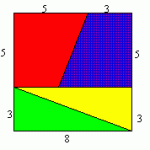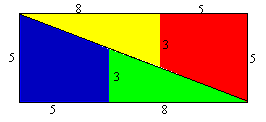Look at this square divided into four pieces : two identical triangles and two identical trapezia.

The edge of length 3 from each triangle matches with the edge of length 3 from a trapezium so that the four pieces from the square now occupy a rectangular space.

The square had side length 8 and area 64.

The area of the rectangle is 65, that is 13 by 5 square units. Can the area have changed?

Can you explain what has happened?

AIMSSEC Notes for Teachers – Doesn’t Add Up

1.Tau Tamako says: